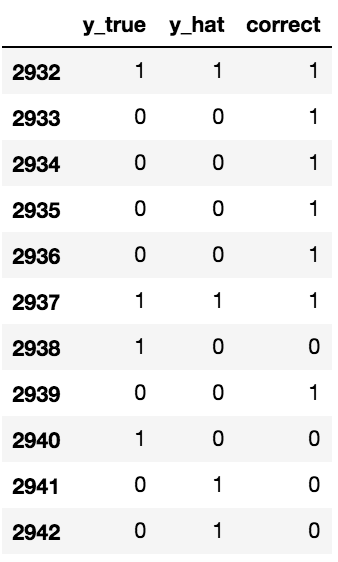# Examining the model performance

We'll start by making predictions on our test data and then we'll examine whether our predictions were correct:

```y_hat = clf.predict(X_test)
y_true = y_test

pdf = pd.DataFrame({'y_true': y_true, 'y_hat': y_hat})

pdf['correct'] = pdf.apply(lambda x: 1 if x['y_true'] == x['y_hat'] else 0, axis=1)

pdf ```

The preceding code generates the following output:Let's now look at what percentage of the 200 IPOs in our test dataset we should have invested in—remember, that means they rose over 2.5% from the open to the close:

`pdf['y_true'].value_counts(normalize=True) `

The preceding code generates the following output:

Get Python Machine Learning Blueprints - Second Edition now with the O’Reilly learning platform.

O’Reilly members experience books, live events, courses curated by job role, and more from O’Reilly and nearly 200 top publishers.